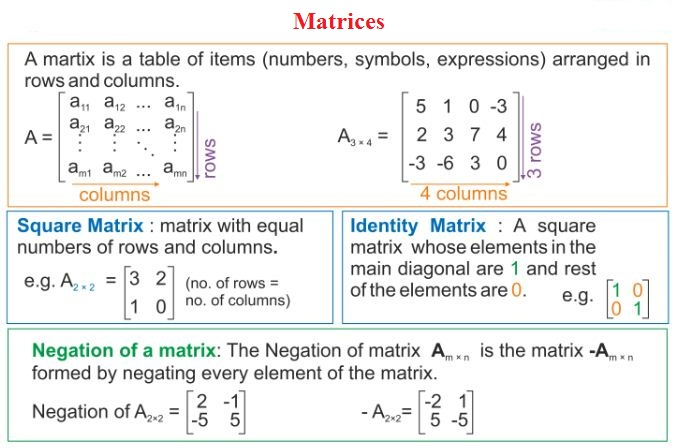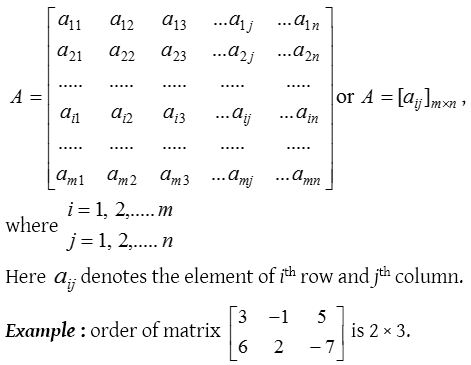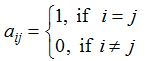## Matrices

Definition
A rectangular arrangement of numbers (which may be real or complex numbers) in rows and columns, is called a matrix. This arrangement is enclosed by small ( ) or big [ ] brackets. The numbers are called the elements of the matrix or entries in the matrix.### Order of a matrix

A matrix having m rows and n columns is called a matrix of order m×n or simply m×n matrix (read as an m by n matrix). A matrix A of order m×n is usually written in the following manner:A matrix of order m × n contains mn elements. Every row of such a matrix contains n elements and every column contains m elements.

### Equality of matrices

Two matrix A and B are said to be equal matrix if they are of same order and their corresponding elements are equal.

### Types of matrices

1. Row matrix: A matrix is said to be a row matrix or row vector if it has only one row and any number of columns. Example: [5 0 3] is a row matrix of order 1× 3 and  is a row matrix of order 1×1.
2. Column matrix: A matrix is said to be a column matrix or column vector if it has only one column and any number of rows.
Example: $$\left[ \begin{matrix} 2 \\ 3 \\ -6 \end{matrix} \right]$$ is a column matrix of order 3 × 1 and  is a column matrix of order 1 × 1. Observe that  is both a row matrix as well as a column matrix.
3. Singleton matrix: If in a matrix there is only one element then it is called singleton matrix.
Thus, A = [aij]m×n is a singleton matrix, if m = n =1
Example: , , [a], [–3] are singleton matrices.
4. Null or zero matrix: If in a matrix all the elements are zero then it is called a zero matrix and it is generally denoted by O. Thus A = [aij]m×n is a zero matrix if aij = 0 for all i and j.
Example: $$\left[ 0 \right] ,\begin{bmatrix} 0 & 0 \\ 0 & 0 \end{bmatrix},\left[ \begin{matrix} 0 & 0 & 0 \\ 0 & 0 & 0 \end{matrix} \right] ,\left[ \begin{matrix} 0 & 0 \end{matrix} \right]$$ are all zero matrices, but of different orders.
5. Square matrix: If number of rows and number of columns in a matrix are equal, then it is called a square matrix.
Thus A = [aij]m×n is a square matrix if m = n.
Example: $$\left[ \begin{matrix} { a }_{ 11 } & { a }_{ 12 } & { a }_{ 13 } \\ { a }_{ 21 } & { a }_{ 22 } & { a }_{ 23 } \\ { a }_{ 31 } & { a }_{ 32 } & { a }_{ 33 } \end{matrix} \right]$$ is a square matrix of order 3×3.
(i) If m ≠ n then matrix is called a rectangular matrix.
(ii) The elements of a square matrix A for which i = j, i.e. a11, a22, a33, ….. amn are called diagonal elements and the line joining these elements is called the principal diagonal or leading diagonal of matrix A.
6. Diagonal matrix: If all elements except the principal diagonal in a square matrix are zero, it is called a diagonal matrix. Thus a square matrix A = [aij] is a diagonal matrix if aij ≠ 0 when i ≠ j.
Example: $$\left[ \begin{matrix} 2 & 0 & 0 \\ 0 & 3 & 0 \\ 0 & 0 & 4 \end{matrix} \right]$$ is a diagonal matrix of order 3× 3, which can be denoted by diag [2, 3, 4].
7. Identity matrix: A square matrix in which elements in the main diagonal are all ‘1’ and rest are all zero is called an identity matrix or unit matrix. Thus, the square matrix A = [aij] is an identity matrix, ifWe denote the identity matrix of order n by In.
Example: $$\left[ 1 \right] ,\begin{bmatrix} 1 & 0 \\ 0 & 1 \end{bmatrix},\left[ \begin{matrix} 1 & 0 & 0 \\ 0 & 1 & 0 \\ 0 & 0 & 1 \end{matrix} \right]$$ are identity matrices of order 1, 2 and 3 respectively.
8. Scalar matrix: A square matrix whose all non diagonal elements are zero and diagonal elements are equal is called a scalar matrix. Thus, if A = [aij] is a square matrix and, then A is a scalar matrix.
Unit matrix and null square matrices are also scalar matrices.
9. Triangular matrix: A square matrix [aij] is said to be triangular matrix if each element above or below the principal diagonal is zero. It is of two types
(i) Upper triangular matrix: A square matrix [aij] is called the upper triangular matrix, if aij = 0 when i > j.
Example: $$\left[ \begin{matrix} 3 & 1 & 2 \\ 0 & 4 & 3 \\ 0 & 0 & 6 \end{matrix} \right]$$ is an upper triangular matrix of order 3 × 3.
(ii) Lower triangular matrix: A square matrix [aij] is called the lower triangular matrix, if aij = 0 when i< j.
Example: $$\left[ \begin{matrix} 1 & 0 & 0 \\ 2 & 3 & 0 \\ 4 & 5 & 2 \end{matrix} \right]$$ is a lower triangular matrix of order 3 × 3.

### Trace of a matrix

The sum of diagonal elements of a square matrix. A is called the trace of matrix A , which is denoted by tr A.Properties of trace of a matrix
Let A = [aii]n×n  and B = [bij]n×n and λ be a scalar

1. tr(λA) = λ tr(A)
2. tr(A – B) = tr(A) – tr(B)
3. tr(AB) = tr(BA)
4. tr(A)= tr(A’) or tr (AT)
5. tr(In) = n
6. tr (0)= 0
7. tr(AB) ≠ trA.trB

### Addition and subtraction of matrices

If A = [aij]m×n  and B = [bij]m×n are two matrices of the same order then their sum A+B is a matrix whose each element is the sum of corresponding elements i.e., A + B = [aij + bij]m×n.
Similarly, their subtraction is defined as A – B = [aij – bij]m×n

Matrix addition and subtraction can be possible only when matrices are of the same order.
Properties of matrix addition : If A, B and C are matrices of same order, then

1. A + B = B + A (Commutative law)
2. (A + B) + C = A + (B + C) (Associative law)
3. A + O = O + A = A, where O is zero matrix which is additive identity of the matrix.
4. A + (–A) = O = (–A) + A , where (–A) is obtained by changing the sign of every element of A, which is additive inverse of the matrix.
5.